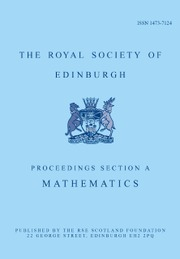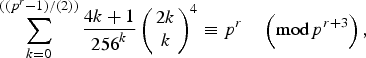Home
Hostname: page-component-6f6fcd54b-pzg5m Total loading time: 0.283 Render date: 2021-05-12T06:22:44.015Z Has data issue: true Feature Flags: {}Proceedings of the Royal Society of Edinburgh Section A: Mathematics

# Some congruences involving fourth powers of central q-binomial coefficients

Published online by Cambridge University Press:  30 January 2019

Corresponding

## Abstract

We prove some congruences on sums involving fourth powers of central q-binomial coefficients. As a conclusion, we confirm the following supercongruence observed by Long [Pacific J. Math. 249 (2011), 405–418]:$$\sum\limits_{k = 0}^{((p^r-1)/(2))} {\displaystyle{{4k + 1} \over {{256}^k}}} \left( \matrix{2k \cr k} \right)^4\equiv p^r\quad \left( {\bmod p^{r + 3}} \right),$$
where p⩾5 is a prime and r is a positive integer. Our method is similar to but a little different from the WZ method used by Zudilin to prove Ramanujan-type supercongruences.

Type
Research Article

## Access options

Get access to the full version of this content by using one of the access options below.

## References

1Andrews, G. E.. The theory of partitions (Cambridge: Cambridge University Press, 1998).Google Scholar
2Babbage, C.. Demonstration of a theorem relating to prime numbers. Edinburgh Philos. J. 1 (1819), 4649.Google Scholar
3Chowla, S., Dwork, B. and Evans, R.. On the mod p 2 determination of$(p-1)/2\choose (p-1)/4$. J. Number Theory (1986), 188196.CrossRefGoogle Scholar
4Gasper, G. and Rahman, M.. Basic hypergeometric series. 2nd Edn, Encyclopedia of Mathematics and its applications, vol. 96 (Cambridge: Cambridge University Press, 2004).10.1017/CBO9780511526251CrossRefGoogle Scholar
5Guo, V. J. W.. Some congruences related to the q-Fermat quotients. Int. J. Number Theory 11 (2015), 10491060.10.1142/S1793042115500554CrossRefGoogle Scholar
6Guo, V. J. W.. Some generalizations of a supercongruence of van Hamme. Integral Transforms Spec. Funct. 28 (2017), 888899.CrossRefGoogle Scholar
7Guo, V. J. W.. q-Analogues of the (E.2) and (F.2) supercongruences of Van Hamme. Ramanujan J. (2018), https://doi.org/10.1007/s11139-018-0021-zGoogle Scholar
8Guo, V. J. W.. A q-analogue of a Ramanujan-type supercongruence involving central binomial coefficients. J. Math. Anal. Appl. 458 (2018), 590600.CrossRefGoogle Scholar
9Koepf, W.. Hypergeometric summation, an Algorithmic approach to summation and special function identities, 2nd Edn (London: Springer, 2014).CrossRefGoogle Scholar
10Liu, J., Pan, H. and Zhang, Y.. A generalization of Morley's congruence. Adv. Differ. Equ. 2015 (2015), 254.10.1186/s13662-015-0568-6CrossRefGoogle Scholar
11Long, L.. Hypergeometric evaluation identities and supercongruences. Pacific J. Math. 249 (2011), 405418.CrossRefGoogle Scholar
12Městrović, R.. Wolstenholme's theorem: its generalizations and extensions in the last hundred and fifty years (1862–2012), preprint, 2011, arXiv:1111.3057.Google Scholar
13Morley, F.. Note on the congruence 24n≡(−1)n (2n)!/(n!)2, where 2n + 1 is a prime. Ann. of Math. 9 (1895), 168170.10.2307/1967516CrossRefGoogle Scholar
14Mortenson, E.. A p-adic supercongruence conjecture of van Hamme. Proc. Amer. Math. Soc. 136 (2008), 43214328.10.1090/S0002-9939-08-09389-1CrossRefGoogle Scholar
15Pan, H.. A q-analogue of Lehmer's congruence. Acta Arith. 128 (2007), 303318.10.4064/aa128-4-1CrossRefGoogle Scholar
16Pan, H.. An elementary approach to$(p-1)/2\choose (p-1)/4$ modulo p 2. Taiwanese J Math. 16 (2012), 21972202.10.11650/twjm/1500406847CrossRefGoogle Scholar
17Petkovšek, M., Wilf, H. S. and Zeilberger, D.. A = B (Wellesley, MA: A K Peters, Ltd., 1996).10.1201/9781439864500CrossRefGoogle Scholar
18Shi, L.-L. and Pan, H.. A q-analogue of Wolstenholme's harmonic series congruence. Amer. Math. Monthly 114 (2005), 529531.10.1080/00029890.2007.11920441CrossRefGoogle Scholar
19Straub, A.. A q-analog of Ljunggren's binomial congruence, 23rd International Conference on Formal Power Series and Algebraic Combinatorics (FPSAC 2011). Discrete Math. Theor. Comput. Sci. Proc., AO, Assoc. Discrete Math. Theor. Comput. Sci., Nancy (2011) 897902.Google Scholar
20Sun, Z.-W.. Products and sums divisible by central binomial coefficients. Electron. J. Combin. 20 (2013), #P9.10.37236/3022CrossRefGoogle Scholar
21Swisher, H.. On the supercongruence conjectures of van Hamme. Res. Math. Sci. (2015) 218.Google Scholar
22Tauraso, R.. Some q-analogs of congruences for central binomial sums. Colloq. Math. 133 (2013), 133143.10.4064/cm133-1-9CrossRefGoogle Scholar
23van Hamme, L.. Some conjectures concerning partial sums of generalized hypergeometric series, p-adic functional analysis (Nijmegen, 1996), Lecture Notes in Pure and Appl. Math.,vol. 192 (New York: Dekker, 1997), 223236.Google Scholar
24Wang, S.-D.. Generalizations of a supercongruence involving$\left( {\matrix{ {2k} \cr k \cr}} \right)^4$. J. Difference Equ. Appl. 24 (2018), 13751383.10.1080/10236198.2018.1485667CrossRefGoogle Scholar
25Wolstenholme, J.. On certain properties of prime numbers. Quart. J. Pure Appl. Math. 5 (1862), 3539.Google Scholar
26Zudilin, W.. Ramanujan-type supercongruences. J. Number Theory 129 (2009), 18481857.CrossRefGoogle Scholar

# Send article to Kindle

Note you can select to send to either the @free.kindle.com or @kindle.com variations. ‘@free.kindle.com’ emails are free but can only be sent to your device when it is connected to wi-fi. ‘@kindle.com’ emails can be delivered even when you are not connected to wi-fi, but note that service fees apply.

Find out more about the Kindle Personal Document Service.

Some congruences involving fourth powers of central q-binomial coefficients
Available formats
×

# Send article to Dropbox

To send this article to your Dropbox account, please select one or more formats and confirm that you agree to abide by our usage policies. If this is the first time you use this feature, you will be asked to authorise Cambridge Core to connect with your <service> account. Find out more about sending content to Dropbox.

Some congruences involving fourth powers of central q-binomial coefficients
Available formats
×

# Send article to Google Drive

To send this article to your Google Drive account, please select one or more formats and confirm that you agree to abide by our usage policies. If this is the first time you use this feature, you will be asked to authorise Cambridge Core to connect with your <service> account. Find out more about sending content to Google Drive.

Some congruences involving fourth powers of central q-binomial coefficients
Available formats
×
×